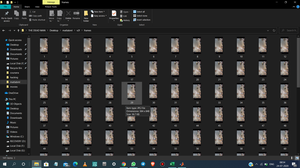Related Articles

# How to Extract Frames From a Video in MATLAB?

• Last Updated : 20 Aug, 2020

Let us see how to extract frames from a video in MATLAB.

Approach :

1. Import the video which is to be converted into frames into the current matlab environment.
2. Extract the total number of frames in the video.
3. Make an empty directory named frames before the execution.
4. Run a for loop and start extracting the frames into the directory.

In our demonstration, we shall consider the following video :

 `% import the video file``obj = VideoReader(``'org.mp4'``);``vid = read(obj);`` ` ` ``% read the total number of frames``frames = obj.NumberOfFrames;`` ` `% file format of the frames to be saved in``ST =``'.jpg'``;`` ` `% reading and writing the frames ``for` `x = 1 : frames`` ` `    ``% converting integer to string``    ``Sx = num2str(x);`` ` `    ``% concatenating 2 strings``    ``Strc = strcat(Sx, ST);``    ``Vid = vid(:, :, :, x);``    ``cd frames`` ` `    ``% exporting the frames``    ``imwrite(Vid, Strc);``    ``cd ..  ``end`

Output :
The frames directory will look something like this :Images saved in the frames folder

My Personal Notes arrow_drop_up